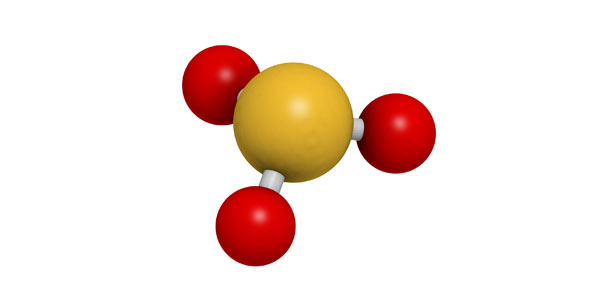# Atoms, Molecules, Elements, Compounds Quiz Questions

14 Questions | Total Attempts: 403SettingsThe atom is the smallest particle of an element. The atoms make up molecules, which in turn makeup elements. In the chemistry classes this week we discussed all the three and a test on this is moving closer. Use the quiz to polish up what you know so far. All the best!

• 1.
Which drawing represents a correct Bohr's Model?
• A.

A

• B.

B

• C.

C

• 2.
How many neutrons are in tin?
• 3.
How many electrons are in tin?
• 4.
What is the atomic number for tin?
• 5.
How many protons are in tin?
• 6.
What is the atomic mass for tin?
• 7.
Which class of elements is to the left of the zig-zag line on the periodic table of elements?
• A.

Metals

• B.

Non-metals

• C.

Metalloids

• 8.
Draw a Bohr Model for the element boron (B).  (Do not type anything into the answer box.  Draw this on your own paper and check it against the answer after you press "Next".)
• 9.
How many electrons are in fluorine?
• 10.
How many neutrons are in fluorine?
• 11.
What is the atomic number for fluorine?
• 12.
How many protons are in fluorine?
• 13.
What is the atomic mass for fluorine?
• 14.
Which class of element is sulfer (S)?
• A.

Metal

• B.

Non-metal

• C.

Metalloid

Related TopicsBack to top